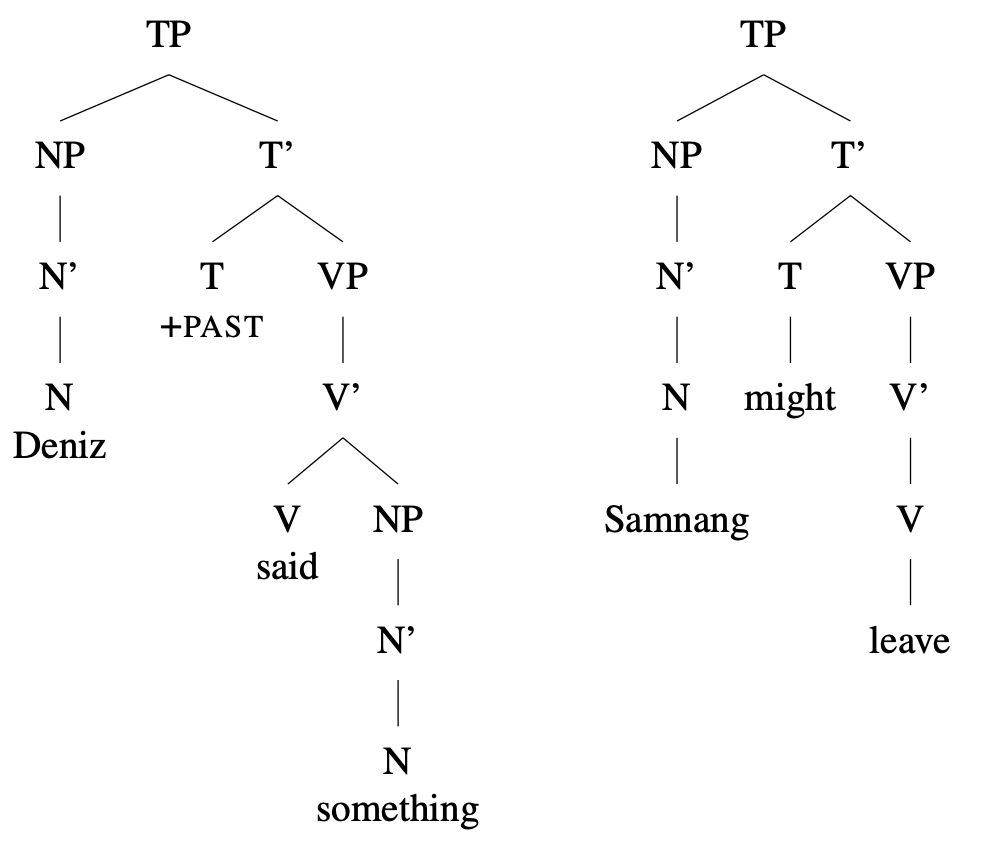# 6.18: Trees- Embedded clauses

$$\newcommand{\vecs}{\overset { \rightharpoonup} {\mathbf{#1}} }$$ $$\newcommand{\vecd}{\overset{-\!-\!\rightharpoonup}{\vphantom{a}\smash {#1}}}$$$$\newcommand{\id}{\mathrm{id}}$$ $$\newcommand{\Span}{\mathrm{span}}$$ $$\newcommand{\kernel}{\mathrm{null}\,}$$ $$\newcommand{\range}{\mathrm{range}\,}$$ $$\newcommand{\RealPart}{\mathrm{Re}}$$ $$\newcommand{\ImaginaryPart}{\mathrm{Im}}$$ $$\newcommand{\Argument}{\mathrm{Arg}}$$ $$\newcommand{\norm}{\| #1 \|}$$ $$\newcommand{\inner}{\langle #1, #2 \rangle}$$ $$\newcommand{\Span}{\mathrm{span}}$$ $$\newcommand{\id}{\mathrm{id}}$$ $$\newcommand{\Span}{\mathrm{span}}$$ $$\newcommand{\kernel}{\mathrm{null}\,}$$ $$\newcommand{\range}{\mathrm{range}\,}$$ $$\newcommand{\RealPart}{\mathrm{Re}}$$ $$\newcommand{\ImaginaryPart}{\mathrm{Im}}$$ $$\newcommand{\Argument}{\mathrm{Arg}}$$ $$\newcommand{\norm}{\| #1 \|}$$ $$\newcommand{\inner}{\langle #1, #2 \rangle}$$ $$\newcommand{\Span}{\mathrm{span}}$$$$\newcommand{\AA}{\unicode[.8,0]{x212B}}$$

In Section 6.6 we observed that complementizers allow clauses to be embedded—that is, to be complements of a verb.

Following our principles of X-bar structure, this means that the complementizer (C) must project a CP. Because verbs can select whether they take an embedded clause, this CP should be the complement of the verb, and should take TP as its own complement.

Recall the sentences we looked at in Section 6.6:

 (1) Deniz said something. (2) Samnang might leave.

Based on the principle of X-bar theory we have seen in previous sections, these would correspond to the tree structures in Figure 6.24.Figure 6.24 Tree diagrams for two TP clauses, [Deniz said something.] and [Samnang might leave.]

Now we can ask: how do these two clauses fit together when (2) is embedded below the verb said of (1), as in the complex sentence in (3)?

 (3) Deniz said that [Samnang might leave].

In addition to what we said in previous sections about the fact that some verbs select CPs as their complements, here’s no space to put the C head, or the CP it’s part of, within either the embedded TP to the main clause VP. Instead, we put the TP inside the CP, as in the tree in Figure 6.25.Figure 6.25 Tree diagram for an embedded clause structure. The embedded clause is a CP that is the sibling of the main clause V

What if the complementizer [C that ] is missing, as in (4)?

 (4) Deniz said that [Samnang might leave].

In this case we’d wouldn’t say that there’s no CP, but that in English the C head can be null. (In Section 6.19 we’ll see other cases where we assume there is an empty C head, because its specifier position is filled.) The tree for (4) would be just like the tree in Figure 6.25, but with no word in the C head.

In Section 6.6 we saw that embedded Yes-No questions are structurally similar to embedded declaratives, but with the complementizer if or whether. Since they have similar structures, their trees are also fundamentally the same, with if or whether occurring in a C head. Thus the tree for a sentence like (5) is as in Figure 6.26.

 (5) Eryl wonders if [ghosts exist].Figure 6.26 Tree diagram showing an embedded Yes-No question. The CP headed by a +Q C is the sibling of the main clause V

In 6.6 Clausal embedding we introduced the feature [–Q] for declarative complementizers and [+Q] for interrogative complementizers. Reframing this in terms of C heads, both that and ∅ are declarative C heads with a [–Q] feature, while both if and whether are interrogative C heads with a [+Q] feature. Verbs select whether they compose with [–Q] CPs, [+Q] CPs, both, or neither.

Finally, in section 6.6 we also introduced embedded nonfinite clauses. The complementizer in these clauses is either ∅ or for, which we could call [–FIN] complementizers. They also differ in that the head of T is the nonfinite marker to.

 (6) I want for [ghosts to exist].Figure 6.27 Tree diagram for an embedded nonfinite clause. The CP headed by -FIN C is the sibling of the main clause V; the embedded TP is headed by to

We’ve now finished formalizing the basic structure of clauses in X-bar theory. Our next step will be to look at how we can formalize transformations in tree structures, introducing the concept of syntactic movement.